# 5.7 Inverses and radical functions  (Page 6/7)

 Page 6 / 7

$f\left(x\right)=\sqrt{6x-8}+5$

$f\left(x\right)=9+2\sqrt{x}$

${f}^{-1}\left(x\right)={\left(\frac{x-9}{2}\right)}^{3}$

$f\left(x\right)=3-\sqrt{x}$

$f\left(x\right)=\frac{2}{x+8}$

${f}^{-1}\left(x\right)={\frac{2-8x}{x}}^{}$

$f\left(x\right)=\frac{3}{x-4}$

$f\left(x\right)=\frac{x+3}{x+7}$

$\text{\hspace{0.17em}}{f}^{-1}\left(x\right)=\frac{7x-3}{1-x}$

$f\left(x\right)=\frac{x-2}{x+7}$

$f\left(x\right)=\frac{3x+4}{5-4x}$

$\text{\hspace{0.17em}}{f}^{-1}\left(x\right)=\frac{5x-4}{4x+3}$

$f\left(x\right)=\frac{5x+1}{2-5x}$

${f}^{-1}\left(x\right)=\sqrt{x+1}-1$

${f}^{-1}\left(x\right)=\sqrt{x+6}+3$

## Graphical

For the following exercises, find the inverse of the function and graph both the function and its inverse.

$f\left(x\right)={x}^{2}+2,\text{\hspace{0.17em}}x\ge 0$

$f\left(x\right)=4-{x}^{2},\text{\hspace{0.17em}}x\ge 0$

${f}^{-1}\left(x\right)=\sqrt{4-x}$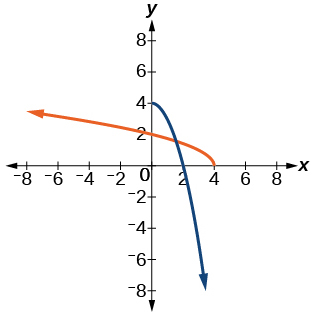$f\left(x\right)={\left(x+3\right)}^{2},\text{\hspace{0.17em}}x\ge -3$

$f\left(x\right)={\left(x-4\right)}^{2},\text{\hspace{0.17em}}x\ge 4$

${f}^{-1}\left(x\right)=\sqrt{x}+4$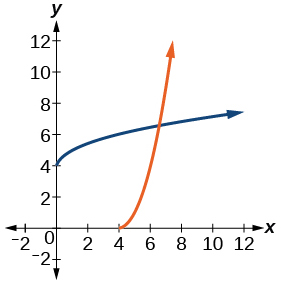$f\left(x\right)={x}^{3}+3$

$f\left(x\right)=1-{x}^{3}$

${f}^{-1}\left(x\right)=\sqrt{1-x}$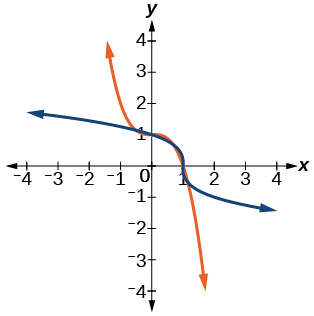$f\left(x\right)={x}^{2}+4x,\text{\hspace{0.17em}}x\ge -2$

$f\left(x\right)={x}^{2}-6x+1,\text{\hspace{0.17em}}x\ge 3$

${f}^{-1}\left(x\right)=\sqrt{x+8}+3$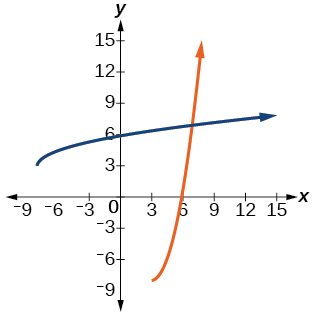$f\left(x\right)=\frac{2}{x}$

$f\left(x\right)=\frac{1}{{x}^{2}},\text{\hspace{0.17em}}x\ge 0$

${f}^{-1}\left(x\right)=\sqrt{\frac{1}{x}}$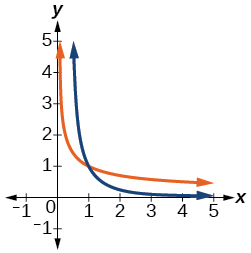For the following exercises, use a graph to help determine the domain of the functions.

$f\left(x\right)=\sqrt{\frac{\left(x+1\right)\left(x-1\right)}{x}}$

$f\left(x\right)=\sqrt{\frac{\left(x+2\right)\left(x-3\right)}{x-1}}$

$\left[-2,1\right)\cup \left[3,\infty \right)$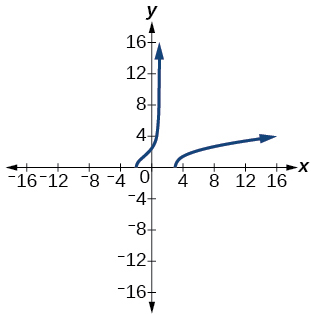$f\left(x\right)=\sqrt{\frac{x\left(x+3\right)}{x-4}}$

$f\left(x\right)=\sqrt{\frac{{x}^{2}-x-20}{x-2}}$

$\left[-4,2\right)\cup \left[5,\infty \right)$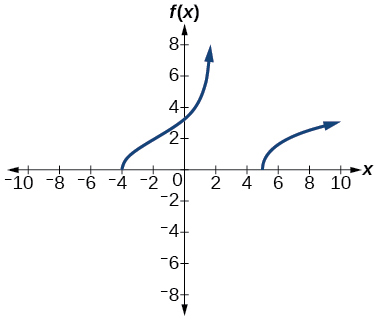$f\left(x\right)=\sqrt{\frac{9-{x}^{2}}{x+4}}$

## Technology

For the following exercises, use a calculator to graph the function. Then, using the graph, give three points on the graph of the inverse with y -coordinates given.

$f\left(x\right)={x}^{3}-x-2,y=1,2,3$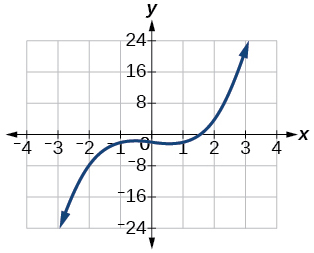$f\left(x\right)={x}^{3}+x-2,y=0,1,2$

$f\left(x\right)={x}^{3}+3x-4,y=0,1,2$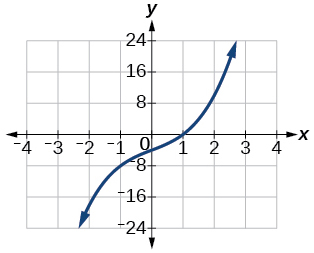$f\left(x\right)={x}^{3}+8x-4,y=-1,0,1$

$f\left(x\right)={x}^{4}+5x+1,y=-1,0,1$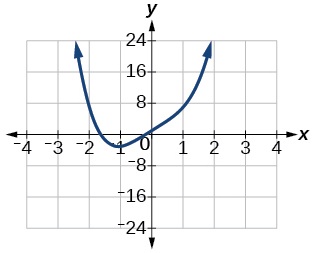## Extensions

For the following exercises, find the inverse of the functions with $\text{\hspace{0.17em}}a,b,c\text{\hspace{0.17em}}$ positive real numbers.

$f\left(x\right)=a{x}^{3}+b$

$f\left(x\right)={x}^{2}+bx$

${f}^{-1}\left(x\right)=\sqrt{x+\frac{{b}^{2}}{4}}-\frac{b}{2}$

$f\left(x\right)=\sqrt{a{x}^{2}+b}$

$f\left(x\right)=\sqrt{ax+b}$

${f}^{-1}\left(x\right)=\frac{{x}^{3}-b}{a}$

$f\left(x\right)=\frac{ax+b}{x+c}$

## Real-world applications

For the following exercises, determine the function described and then use it to answer the question.

An object dropped from a height of 200 meters has a height, $\text{\hspace{0.17em}}h\left(t\right),\text{\hspace{0.17em}}$ in meters after $\text{\hspace{0.17em}}t\text{\hspace{0.17em}}$ seconds have lapsed, such that $\text{\hspace{0.17em}}h\left(t\right)=200-4.9{t}^{2}.\text{\hspace{0.17em}}$ Express $\text{\hspace{0.17em}}t\text{\hspace{0.17em}}$ as a function of height, $\text{\hspace{0.17em}}h,\text{\hspace{0.17em}}$ and find the time to reach a height of 50 meters.

$t\left(h\right)=\sqrt{\frac{200-h}{4.9}},\text{\hspace{0.17em}}$ 5.53 seconds

An object dropped from a height of 600 feet has a height, $\text{\hspace{0.17em}}h\left(t\right),\text{\hspace{0.17em}}$ in feet after $\text{\hspace{0.17em}}t\text{\hspace{0.17em}}$ seconds have elapsed, such that $\text{\hspace{0.17em}}h\left(t\right)=600-16{t}^{2}.\text{\hspace{0.17em}}$ Express $\text{\hspace{0.17em}}t\text{\hspace{0.17em}}$ as a function of height $\text{\hspace{0.17em}}h,\text{\hspace{0.17em}}$ and find the time to reach a height of 400 feet.

The volume, $\text{\hspace{0.17em}}V,\text{\hspace{0.17em}}$ of a sphere in terms of its radius, $\text{\hspace{0.17em}}r,\text{\hspace{0.17em}}$ is given by $\text{\hspace{0.17em}}V\left(r\right)=\frac{4}{3}\pi {r}^{3}.\text{\hspace{0.17em}}$ Express $\text{\hspace{0.17em}}r\text{\hspace{0.17em}}$ as a function of $\text{\hspace{0.17em}}V,\text{\hspace{0.17em}}$ and find the radius of a sphere with volume of 200 cubic feet.

$r\left(V\right)=\sqrt{\frac{3V}{4\pi }},\text{\hspace{0.17em}}$ 3.63 feet

The surface area, $\text{\hspace{0.17em}}A,\text{\hspace{0.17em}}$ of a sphere in terms of its radius, $\text{\hspace{0.17em}}r,\text{\hspace{0.17em}}$ is given by $\text{\hspace{0.17em}}A\left(r\right)=4\pi {r}^{2}.\text{\hspace{0.17em}}$ Express $\text{\hspace{0.17em}}r\text{\hspace{0.17em}}$ as a function of $\text{\hspace{0.17em}}V,\text{\hspace{0.17em}}$ and find the radius of a sphere with a surface area of 1000 square inches.

A container holds 100 mL of a solution that is 25 mL acid. If $\text{\hspace{0.17em}}n\text{\hspace{0.17em}}$ mL of a solution that is 60% acid is added, the function $\text{\hspace{0.17em}}C\left(n\right)=\frac{25+.6n}{100+n}\text{\hspace{0.17em}}$ gives the concentration, $\text{\hspace{0.17em}}C,\text{\hspace{0.17em}}$ as a function of the number of mL added, $\text{\hspace{0.17em}}n.\text{\hspace{0.17em}}$ Express $\text{\hspace{0.17em}}n\text{\hspace{0.17em}}$ as a function of $\text{\hspace{0.17em}}C\text{\hspace{0.17em}}$ and determine the number of mL that need to be added to have a solution that is 50% acid.

$n\left(C\right)=\frac{100C-25}{.6-C},\text{\hspace{0.17em}}$ 250 mL

The period $\text{\hspace{0.17em}}T,\text{\hspace{0.17em}}$ in seconds, of a simple pendulum as a function of its length $\text{\hspace{0.17em}}l,\text{\hspace{0.17em}}$ in feet, is given by $\text{\hspace{0.17em}}T\left(l\right)=2\pi \sqrt{\frac{l}{32.2}}\text{\hspace{0.17em}}$ . Express $\text{\hspace{0.17em}}l\text{\hspace{0.17em}}$ as a function of $\text{\hspace{0.17em}}T\text{\hspace{0.17em}}$ and determine the length of a pendulum with period of 2 seconds.

The volume of a cylinder , $\text{\hspace{0.17em}}V,\text{\hspace{0.17em}}$ in terms of radius, $\text{\hspace{0.17em}}r,\text{\hspace{0.17em}}$ and height, $\text{\hspace{0.17em}}h,\text{\hspace{0.17em}}$ is given by $\text{\hspace{0.17em}}V=\pi {r}^{2}h.\text{\hspace{0.17em}}$ If a cylinder has a height of 6 meters, express the radius as a function of $\text{\hspace{0.17em}}V\text{\hspace{0.17em}}$ and find the radius of a cylinder with volume of 300 cubic meters.

$r\left(V\right)=\sqrt{\frac{V}{6\pi }},\text{\hspace{0.17em}}$ 3.99 meters

The surface area, $\text{\hspace{0.17em}}A,\text{\hspace{0.17em}}$ of a cylinder in terms of its radius, $\text{\hspace{0.17em}}r,\text{\hspace{0.17em}}$ and height, $\text{\hspace{0.17em}}h,\text{\hspace{0.17em}}$ is given by $\text{\hspace{0.17em}}A=2\pi {r}^{2}+2\pi rh.\text{\hspace{0.17em}}$ If the height of the cylinder is 4 feet, express the radius as a function of $\text{\hspace{0.17em}}V\text{\hspace{0.17em}}$ and find the radius if the surface area is 200 square feet.

The volume of a right circular cone, $\text{\hspace{0.17em}}V,\text{\hspace{0.17em}}$ in terms of its radius, $\text{\hspace{0.17em}}r,\text{\hspace{0.17em}}$ and its height, $\text{\hspace{0.17em}}h,\text{\hspace{0.17em}}$ is given by $\text{\hspace{0.17em}}V=\frac{1}{3}\pi {r}^{2}h.\text{\hspace{0.17em}}$ Express $\text{\hspace{0.17em}}r\text{\hspace{0.17em}}$ in terms of $\text{\hspace{0.17em}}h\text{\hspace{0.17em}}$ if the height of the cone is 12 feet and find the radius of a cone with volume of 50 cubic inches.

$r\left(V\right)=\sqrt{\frac{V}{4\pi }},\text{\hspace{0.17em}}$ 1.99 inches

Consider a cone with height of 30 feet. Express the radius, $\text{\hspace{0.17em}}r,\text{\hspace{0.17em}}$ in terms of the volume, $\text{\hspace{0.17em}}V,\text{\hspace{0.17em}}$ and find the radius of a cone with volume of 1000 cubic feet.

#### Questions & Answers

write down the polynomial function with root 1/3,2,-3 with solution
if A and B are subspaces of V prove that (A+B)/B=A/(A-B)
write down the value of each of the following in surd form a)cos(-65°) b)sin(-180°)c)tan(225°)d)tan(135°)
Prove that (sinA/1-cosA - 1-cosA/sinA) (cosA/1-sinA - 1-sinA/cosA) = 4
what is the answer to dividing negative index
In a triangle ABC prove that. (b+c)cosA+(c+a)cosB+(a+b)cisC=a+b+c.
give me the waec 2019 questions
the polar co-ordinate of the point (-1, -1)
prove the identites sin x ( 1+ tan x )+ cos x ( 1+ cot x )= sec x + cosec x
tanh`(x-iy) =A+iB, find A and B
B=Ai-itan(hx-hiy)
Rukmini
what is the addition of 101011 with 101010
If those numbers are binary, it's 1010101. If they are base 10, it's 202021.
Jack
extra power 4 minus 5 x cube + 7 x square minus 5 x + 1 equal to zero
the gradient function of a curve is 2x+4 and the curve passes through point (1,4) find the equation of the curve
1+cos²A/cos²A=2cosec²A-1
test for convergence the series 1+x/2+2!/9x3ByByByByBy